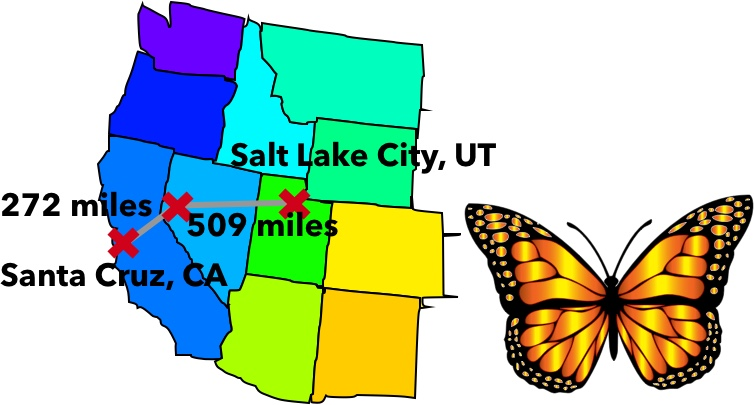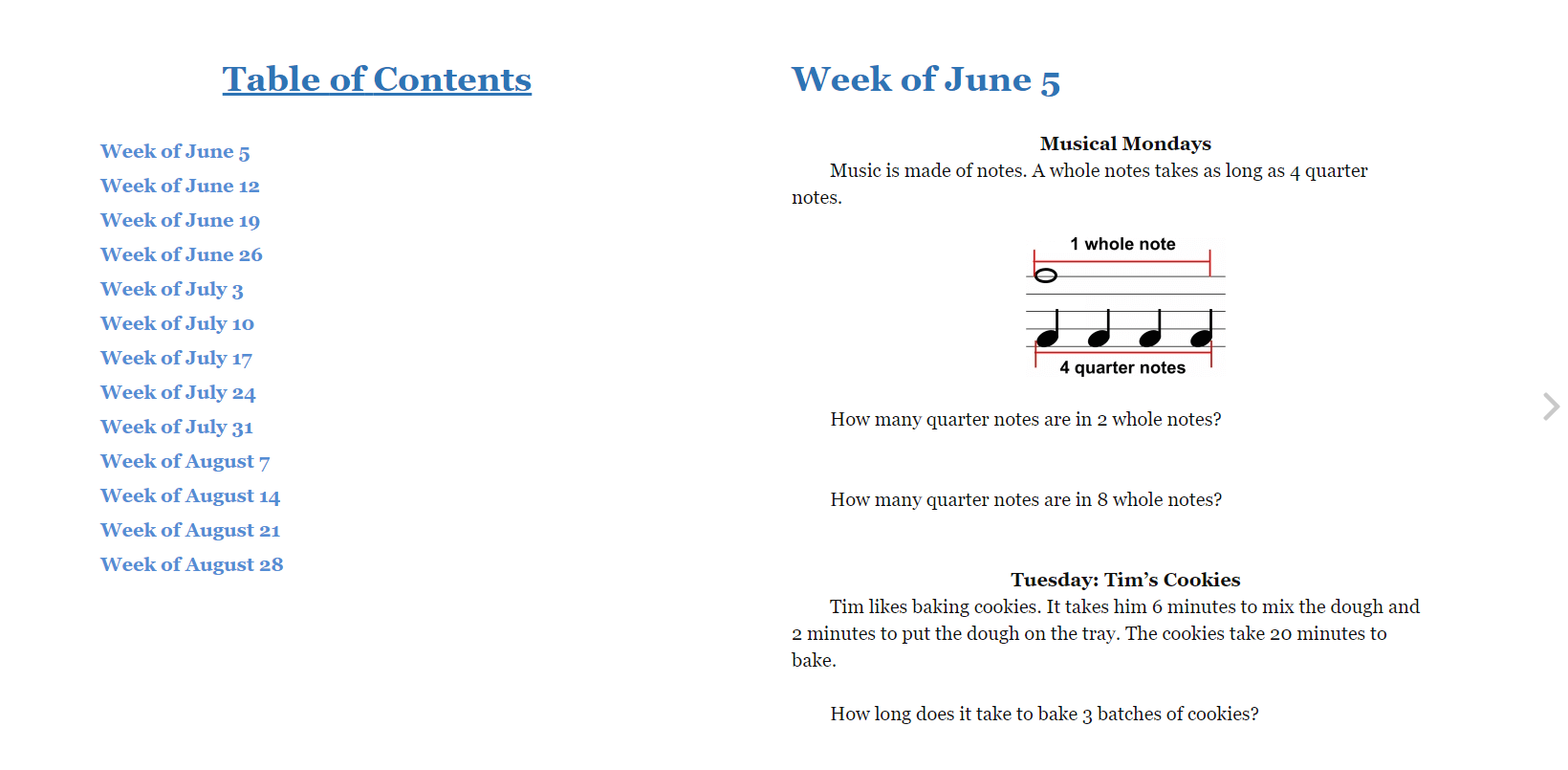# Summer Mathness 4

Welcome to the Summer Mathness 4 page!

You can always buy the book at Amazon.You can enter comments below that link to pictures, YouTube videos and descriptions of your answer and how you found it. Please also support and give good feedback to others working on these problems.

Please note that we will not post our feedback and solutions before the day listed in your book.

Thanks and happy summer!Welcome to the Summer Mathness 4 page! If you are new, please log in first. You can always buy the book at Amazon.You can enter comments below that link to pictures, YouTube videos and descriptions of your answer and how you found it. Please also support and give good feedback to others working on these problems. Please note that we will not post our feedback and solutions before the day listed in your book. Thanks and happy summer! If you need to LOG IN, CLICK HERE first.

## 29 thoughts on “Summer Mathness 4”

1. You could add all three values at once, or add them to at a time.

So, we added 368 + 195 = 563.

We added it in our heads by adding 368 + 200 = 568, and then subtracted 5: 568 – 5 = 563

Then we added 563 + 239 on scratch paper to find the total number of steps: 802

2. There are lots of ways to solve this one, we added the movies first:

. 152 minutes
+ 161 minutes
. 313 minutes

313 minutes is equal to 5 hours and 13 minutes.

5 hours after 1:15 is 6:15. 13 minutes after 6:15 is 6:28.

6:28 p.m. is the earliest that they could finish watching both movies.

3. Whew, Barb does not like this music!!!

They listened to a total of 58+75=133 songs.

Barb liked 5 songs, so she did not like 133-5=128 songs.

4. Solutions for the weekend of June 17-18:

Saturday:
Facts:

• 7 groups of 3 pounds
• Some groups of 5 pounds
• 7 groups + Some groups = 14 groups
• First I know that 7 + 7 = 14, so there must be 7 bags that weigh five pounds.

So the total weight is 7×3+7×5=21+35, which is a total weight of 56 pounds.

Sunday:

The bottom leaf looks longest. It is 5 2/4 inches.

The second leaf looks shortest. It is 4 2/4 inches.

5. Friday (6/16)

27 people into 3 teams requires division: 27 / 3 = 9

I know she needs 27 t-shirts because I just divided 27 by 3, and now I need to multiply the result by 3.

Fact family
27 / 3 = 9
27 / 9 = 3
9 x 3 = 27
3 x 9 = 27

6. Thursday, 6/15:

9 pounds of oranges (3 groups of 3 pounds = 9 pounds)
6 pounds of apples (2 groups of 3 pounds = 6 pounds)
4 pounds of grapes (2 groups of 2 pounds = 4 pounds)
2 pounds of strawberries (2 groups of 1 pound = 2 pounds)

21 total pounds.

Distributing 21 pounds into 3 bags evenly is 21 / 3 = 7 pounds in each of the bags.

7. June has an equal number of Mondays and Wednesdays (we used the calendar app on our phone).

So, we first found the difference (5-3= 2), and then multiplied it by the weeks in June (4). 4×2 = 8 more miles.

8. Tuesday, June 14

386
– 236
150

9. For June 12, Monday, we created a running tally:

Total Minutes: 0, song playing: Dangerous Woman, New Total Minutes: 4 minutes
Total Minutes: 4, song playing: Be Alright, New Total Minutes: 7 minutes

Total Minutes: 7, song playing: Dangerous Woman, New Total Minutes: 11 minutes
Total Minutes: 11, song playing: Be Alright, New Total Minutes: 14 minutes

Total Minutes: 14, song playing: Dangerous Woman, New Total Minutes: 18 minutes
Total Minutes: 18, song playing: Be Alright, New Total Minutes: 21 minutes

Total Minutes: 21, song playing: Dangerous Woman, New Total Minutes: 25 minutes
Total Minutes: 25, song playing: Be Alright, New Total Minutes: 28 minutes

Total Minutes: 28, song playing: Dangerous Woman, New Total Minutes: 32 minutes
Total Minutes: 32, song playing: Be Alright, New Total Minutes: 35 minutes

Total Minutes: 35, song playing: Dangerous Woman, New Total Minutes: 39 minutes
Total Minutes: 39, song playing: Be Alright, New Total Minutes: 42 minutes

Be Alright is playing at minute 40. It was in the middle of the 6th loop, so she can listen to 5 total loops.

10. Here are our answers to the weekend of June 10-11 problems:

For Saturday, we know that the total amount of salad for two days is 6+2+3+6+2+3=22 cups.

If he adds one more cup to two salads, then he will add two more cups of salad.

For Sunday (6/11), we added the liquid together (250+600=850 mL), and then subtracted (1,000-850=150 mL).

And, we could add 250+600 or 600+250 and get the same answer.

11. June 15, Wednesday

There are 4 Mondays and 4 Wednesdays in June (I used a calendar). The difference is 2 miles, then I multiplied by 4 to get 8 miles.

12. Tuesday June 14

I subtracted and found it was 150 miles.

13. Monday, June 13:

The songs are 7 minutes long, so I skip counted:

7, 14, 21, 28, 35, 41

41 minutes is too long, so she can listen 5 complete times.

Since 40 is almost 41, she will be listening to Be Alright

14. Sunday, 6/11:

1,000 – 250 = 750
750 – 600 = 150mL

I could subtract 600, then 250, and get the same final answer.

15. Saturday, 6/10

Each salad has 6+2+3=11 cups. So in 2 days, he makes 2×11=22 cups.

if he adds 1 cup to the salad, then he would add 2 cups in 2 days.

16. We did the chocolates first because there are only 16 so it’s easier. You can see 16 divided by 8 here:

Dividing 64 into 8 equal groups is easy if you share the graham crackers equally in 8 rows.

(Also, you should memorize facts like 16 divided by 8 = 2 and 64 divided by 8 = 8

17. We used a table to organize the data, but afterwards we saw that multiplication:

3 x 2 + 4 x 5 = 26

3+3+4+4+4+4+4=26

Are not too hard also!

18. Thursday (6/8) – I added: 26

19. 6/7/17

For Wed we know that:

1/2 + 1/2 + 1/2 + 1/2 + 1/2 + 1/2 + 1/2 = 7/2 (since 2/2 = 1, we could say 2/2 + 2/2 + 2/2 + 1/2 = 3 1/2)

It’s easier to use multiplication instead of repeated addition:

7 x 1/2 = 7/2

For the whole month, we used a calendar to find there are 30 days in June. Adding 1/2 30 times seemed too long, so we used multiplication:

30 x 1/2 = 15

20. 6/7 for Wed I got 3 1/2 and 15 miles. I used 7/2 and 30/2

21. On wed I got 7/2. Put I couldn’t figure out part 2

1. Where could you find how many days are in a month? You know how to find 7 halves well! Very close!!!

22. For Tuesday, we used a timeline to figure out the long way: